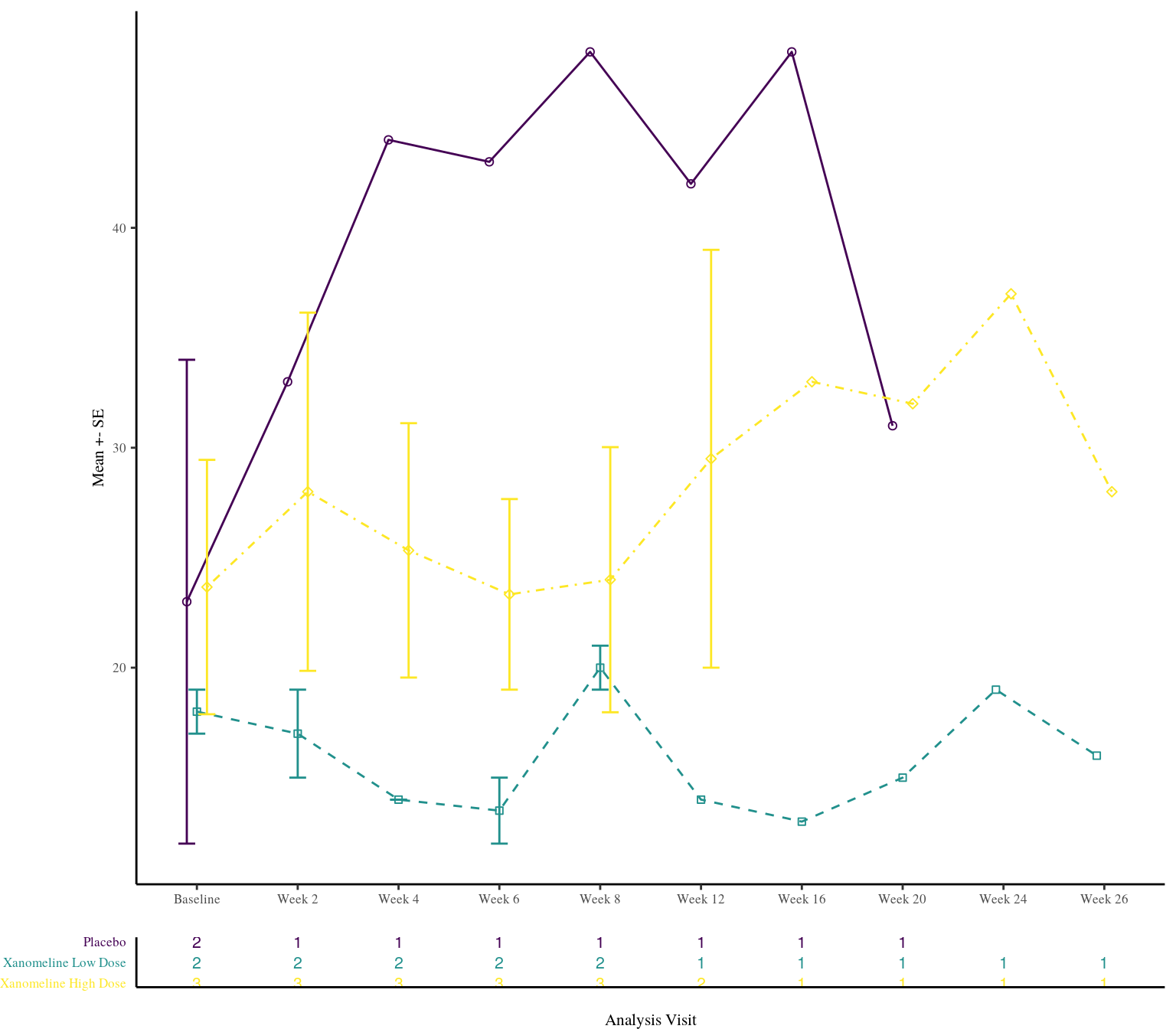This vignette focuses on the visualization functionalities available within the `inTextSummaryTable` package.

If you need to see figures bigger, just click on a graphic, and it will pop up more readable.

Summary statistics can be visualized by:

1. extracting the summary statistics table with the `computeSummaryStatisticsTable` function (used for `getSummaryStatistics` function)
2. visualizing the summary statistics via the `subjectProfileSummaryPlot` function

By default the `subjectProfileSummaryPlot` plots the mean values with error bars based on standard errors.

Moreover, the `subjectProfileSummaryPlot` allows the possibility to add a table of counts of the number of subject for a specific combination of variables.

This vignette will guide the user to

• default visualizations for A4 and presentation documents
• customize palettes of colors, shape, linetypes
• visualization with and without table of counts and by facets
• general customizable input

However, in order to get a full overview of the functionalities the documentation is available in the console with `? subjectProfileSummaryPlot`.

We will first create example data sets to show how the exporting functionalities work. The data sets used here are available in the `clinUtils` package.

# 1 Load packages and data

``````library(inTextSummaryTable)
library(clinUtils)
library(pander)``````
``````# load example data

labelVars <- attr(dataAll, "labelVars")``````

# 2 Compute summary statistics

Below we compute summary statistics for the laboratory data set.

``````dataLB <- subset(dataAll\$ADLBC, grepl("Baseline|Week", AVISIT))
dataLB\$AVISIT <- with(dataLB, reorder(trimws(AVISIT), AVISITN))
dataLB\$TRTA <- with(dataLB, reorder(TRTA, TRTAN))

summaryTableDf <- computeSummaryStatisticsTable(
data = dataLB,
var = "AVAL",
rowVar = c("PARCAT1", "PARAM"),
colVar = c("TRTA", "AVISIT")
)``````

# 3 Visualization for A4 document

The code below shows the default visualization, which plots the mean with standard errors suitable for an A4 document, e.g. Word/pdf.

Note that the table below the plot is based on the counts for treatment and visit variables, as specificed in the `colVar` argument of the code chuck above for `computeSummaryStatisticsTable`.

``````# create the plot
dataPlot <- subset(
summaryTableDf,
!isTotal &
PARAM == "Alanine Aminotransferase (U/L)"
)

subjectProfileSummaryPlot(
data = dataPlot,
xVar = "AVISIT",
colorVar = "TRTA",
labelVars = labelVars,
useLinetype = TRUE,
tableText = "statN"
)``````# 4 Visualization for presentation

The code below shows the default visualization suitable for a presentation, either in Powerpoint or ioslides.

``````subjectProfileSummaryPlot(
data = dataPlot,
xVar = "AVISIT",
colorVar = "TRTA",
labelVars = labelVars,
useLinetype = TRUE,
tableText = "statN",
style = "presentation"
)``````